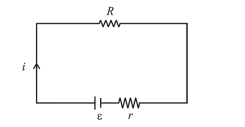# A cell of internal resistance r drives current through an external resistance R.Question:

A cell of internal resistance $r$ drives current through an external resistance $R$. The power delivered by the cell to the external resistance will be maximum when :

1. (1) $\mathrm{R}=0.001 r$

2. (2) $\mathrm{R}=1000 \mathrm{r}$

3. (3) $\mathrm{R}=2 r$

4. (4) $\mathrm{R}=r$

Correct Option: , 4

Solution:

(4) $i=\left(\frac{\varepsilon}{R+r}\right)$

Power delivered to $\mathrm{R}$.

$P=i^{2} R=\left(\frac{\varepsilon}{R+r}\right)^{2} R$$\mathrm{P}$ to be maximum, $\frac{d P}{d R}=0$

or $\frac{d}{d R}\left[\left(\frac{\varepsilon}{R+r}\right)^{2} R\right]=0$

or $R=r$